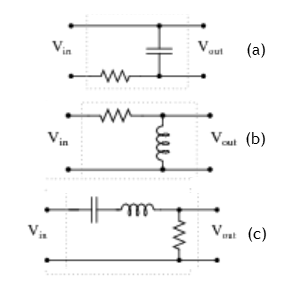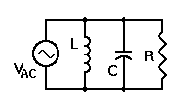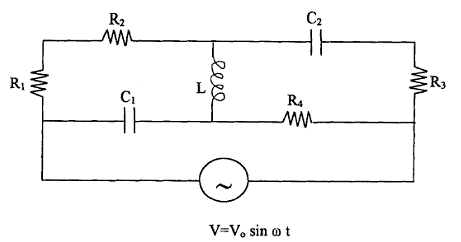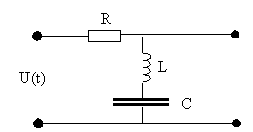### RLC circuits (AC)

#### Problem:

A series RLC circuit is driven by a generator with an emf amplitude of 80 V and a current amplitude of 1.25 A.  The current leads the emf by 0.65 rad.  What are the impedance and the resistance of the circuit?

Solution:

• Concepts:
AC circuits
• Reasoning:
We have a series RLC circuit and a AC generator, generating a sinusoidal voltage.
• Details of the calculation:
In general: V = IZ
Z = R + iωL + 1/(iωC) = R + i(ωL - 1/(ωC)) = (R2 + (ωL - 1/(ωC))2)½exp(iφ)
φ = tan-1((ωL - 1/(ωC))/R)
All that is needed for this problem:
Z = R + iX.
Given:  V = 80 V exp(iωt), I = 1.25 A exp(i(ωt + 0.65)),
Z = V/I = 64 Ω exp(-i0.65),  R =  64 Ω cos(0.65) = 50.95 Ω.

#### Problem:

In the circuit below a 30 V (peak) AC source at 60 Hz is connected to a 90 Ω resistor, a 50 μF capacitor, and a 60 mH inductor in series.  Find the phase of the current through the circuit with respect to the voltage across the source.Solution:

• Concepts:
AC circuits
• Reasoning:
We are asked to analyze an AC circuit.
• Details of the calculation:
V = IZ.
Z = R + iωL + 1/(iωC) = [90 + i*22.6 - i*53)] Ω.
= [90 - i*30.4] Ω = 95 exp(iφ) Ω with φ = -0.326.
I = V/Z = (30 V)/(95 exp(-i0.326)) Ω = 0.316 A exp(i0.326).
The peak value of the current is 0.316 A.
φI - φV = 0.326.  I(t) leads V(t).

#### Problem:

For the circuits shown below, Vin = V0exp(iωt).
In terms of R L and C find Vout and identify which type of filter each circuit represents.Solution:

• Concepts:
Filter circuits
• Reasoning:
We are asked to analyze 3 different AC filter circuits.
• Details of the calculation:
(a)  I = Vin/(ZC + ZR),  Vout = IZC = VinZC/(ZC + ZR).
ZC/(ZC + ZR) = 1/(1 + iRωC) = (1 - iRωC)/(1 + ω2R2C2) = [1/(1 + ω2R2C2)½]e-iφ.
tanφ = RωC.
Vout = V0[1/(1 + ω2R2C2)½]ei(ωt-φ).
Vout --> 0 as ω --> ∞,  Vout --> V0 as ω --> 0, we have a low-pass filter.

(b)   I = Vin/(ZL + ZR),  Vout = IZL = VinZL/(ZL + ZR).
ZL/(ZL + ZR) = ωLexp(iπ/2)/(R + iωL) = exp(iφ)ωL/(R2 + (ωL)2)½.
Vout = V0[ωL/(R2 + (ωL)2)½]ei(ωt+φ).
Vout --> 0 as ω --> 0,  Vout --> V0 as ω --> ∞, we have a high-pass filter.

(c)   I = Vin/(Zc + ZL + ZR),  Vout = IZR = VinZR/(ZC + ZL + ZR).
ZR/(ZC + ZL + ZR) = exp(iφ) R/(R2 + (ωL - 1/(ωC))2)½.
Vout = V0[R/(R2 + (ωL - 1/(ωC))2)½]ei(ωt+φ).
Vout = V0 when ω = 1/(LC)½.
Vout --> 0 as ω --> 0 and as as ω --> ∞, we have a band-pass filter.

#### Problem:

Find the currents in each arm of the parallel LRC circuit with VAC = Re(V0eiωt).   Find the total current and the average power drawn from the generator.Solution:

• Concepts:
AC circuits
• Reasoning:
We have a parallel RLC circuit and a AC generator, generating a sinusoidal voltage.
• Details of the calculation:
Let V = V0eiωt.
IL = Re(V/ZL) = Re(V/iωL) = Re((V0/(ωL))ei(ωt - π/2)) = (V0/(ωL))cos(ωt - π/2)
= (V0/(ωL))sin(ωt).
IC = Re(V/Zc) = Re(ViωC) = Re(V0ωC ei(ωt + π/2) = V0ωC cos(ωt + π/2)
= -V0ωC sin(ωt).
IR = Re(V/R) = Re((V0/R)eiωt) = (V0/R)cos(ωt).
Itot = V0 [(1/(ωL) -  ωC)sin(ωt) + (1/R)cos(ωt)].
Pavg = ½V02/R,  there is no energy dissipated in the reactive part.

#### Problem:

For the circuit shown below, determine the value of the current output through the AC generator, in terms of the symbols given, for two limiting conditions.
(a)  The frequency of the AC generator approaches zero.
(b)  The frequency of the AC generator approaches infinity.Solution:

• Concepts:
AC circuits
• Reasoning:
We have a two-terminal network and a AC generator, generating a sinusoidal voltage.
• Details of the calculation:
Assume V(t) and I(t), are  proportional to exp(iωt).  Assume idealized circuit elements.  Define the impedance Z as Z = V/I.  Then
Z(capacitance) = ZC = 1/(iωC),
Z(inductance) = iωL,
Z(resistance) = ZR = R.
(a)  As ω approaches zero the inductance becomes a short circuit and the capacitors become open circuit elements.  Then the circuit is just a simple loop with 3 resistors in series.  I = V0 exp(iωt)/(R1+R2+R4).
(b)  As ω approaches infinity the capacitors become a short circuits and the inductor becomes an open circuit element.  The circuit then contains 2 loops in parallel, one with resistance R4 and one with the resistors R1 R2 and R3 in series.  The current through the AC generator is I = V0 exp(iωt)(1/(R1+R2+R3) + 1/R4).

#### Problem:

On the input of the RLC filter shown below the periodic voltage oscillating as U(t) = A sin4(ωt) is applied.  Calculate the output voltage after all transients have decayed if the elements R, L, C have been chosen such that  4ω2LC = 1 and  ωRC = 2.Solution:

• Concepts:
AC circuits, Fourier series, the principle of superposition
• Reasoning:
U = ZI for a sinusoidally varying voltage.
• Details of the calculation:
sin4(x) = (1/8)cos(4x) - ½cos(2x) + 3/8.
Use complex notation, the real part of the expression describes the physical quantity.
U(t) = (A/8)exp(i4ωt) + (A/2)exp(i2ωt) + 3A/8 = U1 + U2 + U3.
Use the principle of superposition.
For each of the 3 terms in the Fourier series:  Ui = IZi, Ui' = IZi', Ui' = UiZi'/Zi.
Here Ui is the input and Ui' the output voltage.
Zi/Zi' = (i(ωiL - 1/(ωiC)) + R)/(i(ωiL - 1/(ωiC))) = 1 - iωiRC/(ωi2LC - 1).
Zi/Zi' = [1 + (ωiRC/(ωi2LC - 1))2]½ exp(-φ), with tanφ = ωiRC/(ωi2LC - 1).
Zi'/Zi = [1 + (ωiRC/(ωi2LC - 1))2] exp(φ).
For ωi = 0, Zi'/Zi = 1.
For ωi = 2ω, Zi'/Zi =  i(4ω2LC - 1)/(i(4ω2LC - 1) + 2ωRC) = 0.
For ωi = 4ω, Zi'/Zi = [1 + (4ωRC/(16ω2LC - 1))2] exp(φ) = [1 + (8/3)2] exp(φ) = 0.3511exp(φ).
U' = 3A/8 + (A/8)exp(i(4ωt + φ) = 3A/8 - 0.3511(A/8)cos(4ωt + φ).
The filter passes only the 4ω component (plus a constant offset).